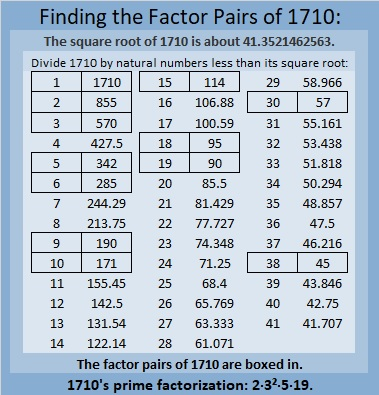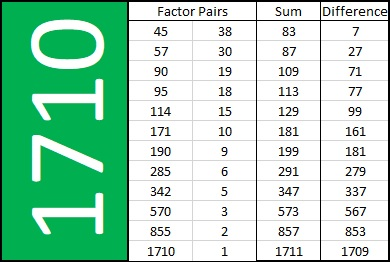# Find the Factor Pairs of 1710. Which One Sums to 181? Which One Subtracts to 181?

Contents

### Today’s Puzzle:

1710 has TWELVE factor pairs. Two of those factor pairs are special. If you add up the factors in one of them, you will get 181, but get this, if you subtract the factors in the other one, you will also get 181. Find the two factor pairs that do that, and you will have essentially solved this puzzle:This Sum-Difference puzzle would be helpful if you had to factor any of these trinomials:
45x² – 181x + 38,
95x² – 181x – 18,
90x² + 181x + 19,
38x² + 181x – 45,

The trinomials are in the form ax² + bx + c, where b = 181, and a·c = ±1710. Whether the 1710 is positive or negative makes all the difference in the world when factoring them!

### Factors of 1710:

• 1710 is a composite number.
• Prime factorization: 1710 = 2 × 3 × 3 × 5 × 19, which can be written 1710 = 2 × 3² × 5 × 19.
• 1710 has at least one exponent greater than 1 in its prime factorization so √1710 can be simplified. Taking the factor pair from the factor pair table below with the largest square number factor, we get √1710 = (√9)(√190) = 3√190.
• The exponents in the prime factorization are 1, 2, 1, and 1. Adding one to each exponent and multiplying we get (1 + 1)(2 + 1)(1 + 1)(1 + 1) = 2 × 3 × 2 × 2 = 24. Therefore 1710 has exactly 24 factors.
• The factors of 1710 are outlined with their factor pair partners in the graphic below.The following chart lists 1710’s factor pairs with their sums and differences:### More About the Number 1710:

1710 is the hypotenuse of a Pythagorean triple:
1026-1368-1710, which is (3-4-5) times 342.

This site uses Akismet to reduce spam. Learn how your comment data is processed.